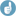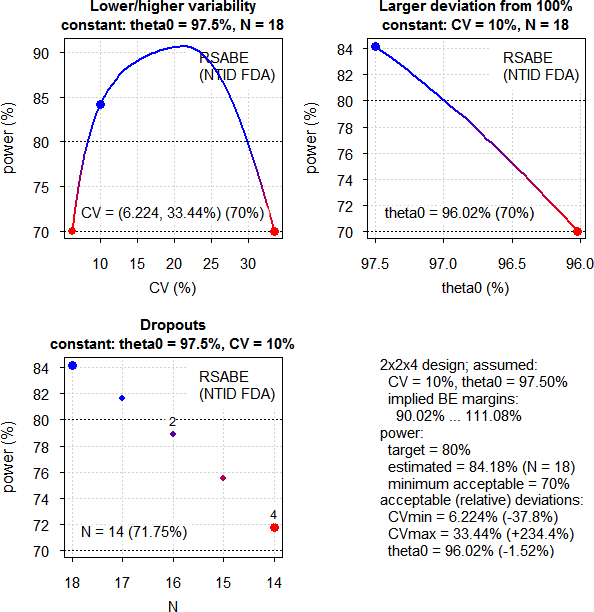narra1813
☆

India,
2019-08-10 09:21

Posting: # 20491
Views: 1,684

## Sample size calculation for NTI molecules [Power / Sample Size]

Dear All,

By using the only power and sample size how we can calculate the N for NTI molecules. what about the other parameters.

Calculating the probability of passing (power) and the sample size (to meet a desired power target) are just two sides of the same coin. One determines the power at a fixed value of n (number of subjects) and the other determines n for a fixed value of power.

FDA published a paper that gives the results of the correct simulations; Jiang W, et al, A Bioequivalence Approach for Generic Narrow Therapeutic Index Drugs: Evaluation of the Reference-Scaled Approach and Variability Comparison Criterion, The AAPS Journal, 17(4), 2015.

Please provide your valuable suggestion on the same, for my understanding the same.

Edit: I deleted another post with identical text. Please follow the Forum’s Policy. I activated the PM-function and edited your profile[Helmut]

Regards

Narra Narendra Babu
Helmut
★★★Vienna, Austria,
2019-08-10 11:23

@ narra1813
Posting: # 20492
Views: 1,426

## PowerTOST: sampleN.NTIDFDA()

Hi Narra,

» By using the only power and sample size how we can calculate the N for NTI molecules. what about the other parameters.

As with any other reference-scaling method we have to use simulations. The conditions for BE of NTIDs are summarized in this post and the statistical method is given in the FDA’s warfarin-guidance.

Sample size estimations are implemented in function sampleN.NTIDFDA() of the R-package PowerTOST since 2013.Example: CVwT = CVwR = 10%, expected T/R-ratio 97.5%, desired (target) power 80%.

library(PowerTOST) sampleN.NTIDFDA(CV = 0.1)

Gives:

+++++++++++ FDA method for NTIDs ++++++++++++            Sample size estimation --------------------------------------------- Study design:  2x2x4 log-transformed data (multiplicative model) 1e+05 studies for each step simulated. alpha  = 0.05, target power = 0.8 CVw(T) = 0.1, CVw(R) = 0.1 True ratio     = 0.975 ABE limits     = 0.8 ... 1.25 Implied scABEL = 0.9002 ... 1.1108 Regulatory settings: FDA - Regulatory const. = 1.053605 - 'CVcap'           = 0.2142 Sample size search  n     power 14   0.717480 16   0.788690 18   0.841790

Note that in this simple call defaults of the function are applied, namely a full replicate 2-sequence 4-period design (TRTR|RTRT), identical within-subject CVs of T and R, desired (target) power 80%, and T/R 97.5% (the FDA requires for NTIDs tighter content specifications).
Let’s explore what happens if T has a higher variability (15%) than R (10%). Abbreviated output.

print(sampleN.NTIDFDA(CV = c(0.15, 0.1), details = FALSE,                       print = FALSE)[8:9], row.names = FALSE) Sample size Achieved power          32        0.81756

Much higher sample size because it will be more difficult to meet the sWT/sWR criterion.

Note that the FDA wants a 4-period full replicate. If you are concerned about blood loss and/or dropouts you may consider a 3-period full replicate (TRT|RTR). Since that deviates from the guidance, I recommend to initiate a controlled correspondence with the OGD first. Like the first example but for 90% power:

sampleN.NTIDFDA(CV = 0.1, design = "2x2x3", targetpower = 0.9) +++++++++++ FDA method for NTIDs ++++++++++++            Sample size estimation --------------------------------------------- Study design:  2x2x3 log-transformed data (multiplicative model) 1e+05 studies for each step simulated. alpha  = 0.05, target power = 0.9 CVw(T) = 0.1, CVw(R) = 0.1 True ratio     = 0.975 ABE limits     = 0.8 ... 1.25 Implied scABEL = 0.9002 ... 1.1108 Regulatory settings: FDA - Regulatory const. = 1.053605 - 'CVcap'           = 0.2142 Sample size search  n     power 34   0.894880 36   0.910210

We can also explore deviations from our assumptions (only equal CVs implemented). For the first example, minimum acceptable power 70% (default of the function):

pa.NTIDFDA(CV = 0.1) Sample size plan RSABE NTID  Design alpha CVwT CVwR theta0 theta1 theta2 Sample size Achieved power Target power   2x2x4  0.05  0.1  0.1  0.975    0.8   1.25          18        0.84179          0.8 Power analysis CV, theta0 and number of subjects which lead to min. acceptable power of at least 0.7:  CV = (0.0622, 0.3344), theta0= 0.9602  N = 14 (power= 0.7175)Note the interesting behavior of power with various CVs. If the CV gets smaller, limits get tighter and power drops. On the other hand, if the CV increases, we have wider limits and gain power. If the CVwR >21.42% the additional criterion “must pass 80–125%” becomes increasingly important and power drops.

We can also explore which of the three criteria are most important. Again the first example and the estimated sample size 18:

power.NTIDFDA(CV = 0.1, n = 18, details = TRUE)   p(BE)  p(BE-sABEc)    p(BE-ABE) p(BE-sratio) 0.84179      0.85628      1.00000      0.97210

The scaled criterion drives the sample size.

The second example where CVwT > CVwR:

power.NTIDFDA(CV = c(0.15, 0.1), n = 32, details = TRUE)   p(BE)  p(BE-sABEc)    p(BE-ABE) p(BE-sratio) 0.81756      0.92270      1.00000      0.87137

Here the sWT/sWR criterion drives the sample size.

» FDA published a paper* …
» Please provide your valuable suggestion on the same, for my understanding the same.

Hope the above helps.

Cheers,
Helmut SchützThe quality of responses received is directly proportional to the quality of the question asked. 🚮
Science QuotesIng. Helmut Schütz